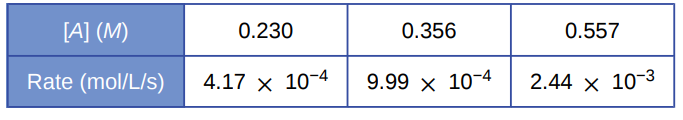# Problem: For the reaction A ⟶ B + C, the following data were obtained at 30 °C:(a) What is the order of the reaction with respect to [A], and what is the rate equation?

###### FREE Expert Solution

We’re being asked to determine the order of the reaction with respect to [A], and what is the rate equation based on the reaction and data given.

Recall that the rate law only focuses on the reactant concentrations and has a general form of:

k = rate constant
A & B = reactants
x & y = reactant orders

We will calculate the rate order first. Since we only have one reactant, we can use any of the data interval given:x = order

86% (19 ratings)###### Problem Details

For the reaction A ⟶ B + C, the following data were obtained at 30 °C:(a) What is the order of the reaction with respect to [A], and what is the rate equation?

Frequently Asked Questions

What scientific concept do you need to know in order to solve this problem?

Our tutors have indicated that to solve this problem you will need to apply the Rate Law concept. You can view video lessons to learn Rate Law. Or if you need more Rate Law practice, you can also practice Rate Law practice problems.

What professor is this problem relevant for?

Based on our data, we think this problem is relevant for Professor Hunter's class at ISU.

What textbook is this problem found in?

Our data indicates that this problem or a close variation was asked in Chemistry - OpenStax 2015th Edition. You can also practice Chemistry - OpenStax 2015th Edition practice problems.